# 量磊C囂冱殻會譜柴利仁永芝栽鹿

+購廣写偬臥心
↙牽旋容呪⦿低珊壓圻勺杭択唖戦堝捲暦匂◦孖壓唖戦堝0.8孵尫扮農杭試強栖晴▷4宰8G二匍堝捲暦匂叙1698圷/3定⇧泣似宸戦羨軸農杭>>>

↙牽旋容呪⦿低珊壓互勺萩利大巷望恂二匍利嫋◦孖壓唖戦堝秀嫋栖晴⇧叙俶500圷/定酔堀喜秀二匍郊利⇧泣似宸戦羨軸農杭>>>

@[toc]

# 1. 嘘尚

## 柴麻字才園殻囂冱

//箭⦿hello world!
#include<stdio.h>
int main()
{
printf("Hello World!\n");//補竃＾Hello World!↙算佩⇄￣哈催坪議何蛍
return 0;
}


↙麻隈⦿厘断峐斑柴麻字恂柴麻⇧枠勣亟竃柴麻議化帶⇧隼朔喘園殻囂冱亟竃栖。柴麻字恂議侭嗤並秤脅頁柴麻◉柴麻議化帶祥頁麻隈。⇄

## 柴麻字議房略圭塀

1) 旦訟隈

2) 屈蛍隈

1) 盾瞥⦿処廁匯倖殻會⇧椎倖殻會辛參編夕尖盾低議殻會⇧隼朔梓孚低議勣箔峇佩
2) 園咎⦿処廁匯倖殻會委低議殻會鍬咎撹字匂囂冱亟議殻會⇧隼朔柴麻字祥辛參岷俊峇佩阻。

## 葎焚担園殻秘壇貫C僥軟◦1) 壓載謹魁栽⇧C囂冱頁率匯議僉夲
2) 孖旗園殻囂冱壓囂隈貧餓呟載弌⇧児云脅才C俊除
3) 囂冱議嬬薦、癖喘糟囃麼勣頁喇 勧由 畳協議。

## C議酒汽煽雰

1969貴伏。
FORTRAN★BCPL★B囂冱★C囂冱

*1967定⇧州播寄僥議 Martin Richards 斤CPL囂冱序佩阻酒晒⇧噐頁恢伏阻BCPL↙Basic Combined Pogramming Language)囂冱。
1970定⇧胆忽唄櫛糞刮片議 Ken Thompson。參BCPL囂冱葎児粥⇧譜柴竃載酒汽拝載俊除啣周議B囂冱↙函BCPL議遍忖銚⇄。旺拝麿喘B囂冱亟阻及匯倖UNIX荷恬狼由。

C囂冱珊鞭欺阻PL/I↙才久蚊狼由載俊除⇧辛參鎮栖恂荷恬狼由吉⇄議唹峒⇧珊才 PDP-II議字匂囂冱嗤載寄購狼
1973/3⇧及眉井議Unix貧竃孖阻C囂冱議園咎匂
1973/11⇧及膨井議Unix頼畠喘C囂冱嶷仟亟議。1989定⇧ANSI窟下阻匯倖炎彈⦿ANSI C
1990定⇧ISO俊鞭阻ANSI議炎彈⦿C89
C議炎彈壓1995定才1999定曾肝厚仟⦿C95才C99。孖壓議園咎匂脅児噐C99阻⇧咀緩云仁殻匆頁。

## 園殻罷周喘焚担

C囂冱議喘余⦿亟荷恬狼由、廼秘塀狼由、駁強殻會↙喘栖駁強U徒⇄、久蚊駁強、夕侘哈陪、夕崧侃尖、蕗咄丼惚↙岷俊公厘断戻工方象議旗鷹音頁喘C囂冱亟議⇄匯乂久蚊議叫廉。
C囂冱頁匯嶽垢匍囂冱⇧喇児粥繁埀聞喘。侭參⦿
**蝕窟丼楕>>僥楼狛殻

C囂冱俶勣瓜園咎嘉嬬塰佩⇧侭參俶勣⦿園辞匂才園咎匂
↙賜宀IDE⇧Integrated Development Environment鹿撹蝕窟桟廠⇧匯倖競践⇄# 2. 秘壇⦿貫Hello World蝕兵

## 及匯倖C殻會

#include<stdio.h>
int main()
{
printf("Hello World!\n");
return 0;
}

**貧極𠮟幣佛催⇧傍苧短嗤隠贋。Ctrl + S 隠贋旺拝芝誼僉夲.c鯉塀。

## 峋盾及匯倖C殻會

#include<stdio.h>
int main()
{

return 0;
}

printf()氏委" "坪議坪否↙忖憲堪⇄圻撃音強仇補竃⇧\n燕幣算佩。

## 酒汽柴麻

printf("%d",23+43);

%d頁媼了憲⇧傍苧朔中氏嗤匯倖屁方補竃欺宸倖了崔貧。

printf("23+43=%d",23+43);

++
--
*
/
%函噫
( )( )凄催

# 3. 延楚

## 延楚協吶

1) 嗤一隈補秘方忖◉
2) 嗤仇圭慧補秘議方忖◉
3) 補秘議方忖歌嚥柴麻。

    int price=0;//協吶阻屁侘延楚price⇧窃侏頁int⇧兜兵峙=0
printf("萩補秘署駆↙圷⇄⦿");
int change=100-price;
printf("孀艇%d圷。\n",change);

<窃侏兆各> <延楚兆各>

↙巴和指概岻念⇧殻會音氏響欺販採叫廉。⇄

## 延楚験峙嚥兜兵晒

    int price=0;//凪嶄=頁験峙塰麻憲⇧委嘔円議峙験公恣円議延楚。

    int a=0,b=1;

C囂冱頁嗤窃侏議囂冱⇧侭嗤延楚壓聞喘念駅倬枠協吶賜蕗苧◉侭嗤延楚駅倬嗤鳩協議方象窃侏↙燕幣壓延楚嶄辛參贋慧焚担劔議方象⇄⇧延楚嶄匆峪嬬贋慧峺協窃侏議方象⇧殻會塰佩狛殻嶄匆音嬬個延延楚議窃侏。
int charge=100-price;

//C囂冱
int price=0;
printf("萩補秘署駆↙圷⇄⦿");
scanf("%d",&price);
int change=100-price;
printf("孀艇%d圷。\n",change);
//勧由議ANSI C峪嬬壓蝕遊議仇圭協吶延楚
int price=0;
int change=0;

printf("萩補秘署駆↙圷⇄⦿");
scanf("%d",&price);
change=100-price;
printf("孀艇%d圷。\n",change);

## 延楚補秘

scanf()痕方⇧響欺議潤惚験峙公朔中議延楚
↙廣吭延楚念議&⇄

## 械楚vs延楚

    const int amount=100;

↙匯違const議延楚畠寄亟⇄
scanf議腎鯉嗤讐梢⇧參朔壅傍。補秘扮泌惚勣曾倖方⇧壓嶄寂、恷朔巴腎鯉賜指概⇧柴麻字響欺曾倖方忖扮唯峭

## 検泣方

C囂冱嶄曾倖屁方恂塰麻誼欺議潤惚匆峪嬬頁倖屁方↙肇渠弌方何蛍⇄。遇10才10.0壓c嶄頁頼畠音揖議曾倖方忖⇧10.0頁検泣方↙検泣方峺弌方泣扮辛參検強議⇧頁柴麻字嶄燕幣蛍方才涙尖方議匯嶽圭塀。繁断喘検泣方栖各柵嗤弌方泣議方⇄↙凪糞珊嗤協泣方。徽頁壓c囂冱嶄涙⇄

↙泌⦿

int a,b;
printf("a/b*3");//個序葎a/b*3.0

(柴麻字戦氏嗤宸嶽歓癌議屁方⇧頁咀葎塰麻酔、媼仇弌。遇拝晩械伏試嶄寄謹匆頁屁方塰麻。)

## 燕器塀int hour1,min1;
int hour2,min2;

scanf("%d %d",&hour1,&min1);
scanf("%d %d",&hour2,&min2);

int t1=hour1*60+min1;//委弌扮廬晒葎蛍嶝汽了
int t2=hour2*60+min2;

int t=t2-t1;
printf("扮寂餓頁%d弌扮%d蛍。＾,t/60,t%60);//t/60頁弌扮何蛍◉t%60函噫⇧頁蛍嶝何蛍

## 塰麻憲單枠雫

↙double補秘議扮昨喘%lf⇧補竃議扮昨喘%f祥佩⇄

1+汽朕音延徭嘔﨑恣a*+b
1-汽朕函減徭嘔﨑恣a*-b
2*徭恣﨑嘔a*b
2/徭恣﨑嘔a/b
2%函噫徭恣﨑嘔a%b
3+徭恣﨑嘔a+b
3-徭恣﨑嘔a-b
4=験峙徭嘔﨑恣a=b
↙a+b,a-b頁褒朕◉+a,-a頁汽朕⇧屢輝噐屎減催⇄

a=6議潤惚祥頁a瓜験嚠議峙⇧匆祥頁6
a=b=6⦿徭嘔﨑恣⇧a=(b=6)。

    result=a=b=3+c;
result=(result=result*2)*6*(result=3+result);

↙C囂冱短嗤竹肝⇧侭參峪嬬匯倖倖核。⇄

## 住算延楚

a=b;
b=a;

c=a;
a=b;
b=c;DEV C++嶄⇧壓恣円方忖椎戦泣似匯和⇧隼朔殻會塰佩扮祥氏壓宸匯佩唯峭。C囂冱⦿聞喘Dev C++僅泣距編

## 鹸栽験峙

a+=5燕幣a=a+5(廣吭曾倖塰麻憲岻寂涙腎鯉)

a*=b+5燕幣a=a*(b+5),泌惚峐枠麻a=a*b芝誼紗凄催⇄
INC弓奐、DEC弓受塰麻憲⦿++--⦿汽朕塰麻憲⇧峪嬬嗤匯倖麻徨⇧宸倖麻徨珊峪嬬頁延楚↙泌a++朔弸侘塀⇧++a念弸侘塀⇄斑乎延楚+1/-1◉
a++塰麻塀議峙頁a+1參念議峙◉++a塰麻塀議峙頁a+1參朔議峙。涙胎陳倖⇧a議峙脅+1阻++--辛參汽鏡聞喘⇧徽音勣委万怏栽序燕器塀⇧鹸墫。

# 4. 登僅↙僉夲潤更⇄

if訳周登僅

    if(訳周){
勣峇佩議囂鞘⇧峪嗤訳周撹羨扮嘉氏峇佩
}

## 登僅議訳周

==登僅屢吉
!=音屢吉

printf("%d\n",5>3);
printf("%d\n",5==3);

(園殻囂冱脅頁辛參繁葎刮屬議)
int r=a>0;枠登僅a頁倦>0,壅委0/1議峙験公r
==!=單枠雫曳凪麿議詰

5>3==6>41==1撹羨
6>5>41>4音撹羨

## 孀巣柴麻匂

flowchat
st=>start: 蝕兵
i1=>inputoutput: 補秘杭択議署駆
i2=>inputoutput: 補秘屶原議同中
o1=>operation: 柴麻孀巣
c=>condition: 登僅噫駆頁倦割怎
o2=>inputoutput: 嬉咫孀巣
o3=>inputoutput: 御岑喘薩噫駆音怎參杭択
e=>end: 潤崩

st->i1->i2->o1->c
c(yes)->o2->e
c(no)->o3->e
    //兜兵晒
int price=0;
int bill=0;
//響秘署駆才同中
printf("萩補秘署駆⦿");
scanf("%d",&price);
printf("萩補秘同中⦿");
scanf("%d",&bill);
//柴麻孀巣

//頁↙汽佩⇄廣瞥⇧委殻會蛍撹阻眉倖何蛍⦿兜兵晒⇧響秘署駆才同中⇧柴麻旺嬉咫孀巣◉
c99辛參⇧ASCII音屶隔
comment斤殻會議孔嬬短嗤販採唹峒⇧徽頁吏吏嬬聞殻會厚否叟瓜繁窃響宀尖盾。
/* */嶄寂辛參嗤謹佩廣瞥。

## 倦夸

else{ }if議温鹿

    int a,b;
printf("萩補秘曾倖屁方⦿");
scanf("%d %d",&a,&b);
int max=0;
if(a>b)max=a;
else max=b;
printf("寄議椎倖頁%d\n",max);

    int a,b;
printf("萩補秘曾倖屁方⦿");
scanf("%d %d",&a,&b);
int max=b;
if(a>b)max=a;
printf("寄議椎倖頁%d\n",max);

## 短嗤寄凄催議if囂鞘

if囂鞘辛參音紗寄凄催

if↙a>b⇄
max=a;

If宸佩潤崩旺短嗤囂鞘潤崩議炎崗;遇朔中議験峙囂鞘亟壓和匯佩拝抹序阻⇧潤崩扮嗤＾◉￣。宸燕苧宸訳験峙囂鞘頁if囂鞘議匯何蛍⇧if囂鞘啜嗤才陣崙宸訳験峙囂鞘⇧畳協麿頁倦勣瓜峇佩。

## 廼耗議if-else

if(a>b)
{
if(a>c)max=a;
else max=c;
}
else
{
if(b>c)max=b;
else max=c;
}


## 雫選議if-else if

flowchat
st=>start: 蝕兵
i=>inputoutput: 補秘
o=>inputoutput: 補竃
c1=>condition: 頁倦諾怎訳周1
c2=>condition: 頁倦諾怎訳周2
o1=>inputoutput: 補竃訳周1
o2=>inputoutput: 補竃訳周2
o3=>inputoutput: 補竃訳周3
en=>end: 潤崩
st->i->c1
c1(yes)->o1->en
c1(no)->c2
c2(yes)->o2->en
c2(no)->o3->en

else
{
if()
else
}

if(x<0)f=-1;
else if(x==0)f=0;
else f=1;
printf("%d",f);
if(x<0)printf("%d",-1);
else if(x==0)printf("%d",0);
else printf("%d",1);

## if-else議械需危列

1. 梨芝亟寄凄催議三⇧訳周撹羨扮峪峇佩朔中匯鞘
2. If朔中涙蛍催
3. ==才=⦿if峪勣箔凄催戦頁0賜掲0
4. 聞繁是雌議else

3.2.4 謹揃蛍屶

switch(print){
case 1:
printf("1");
break;
case 2:
printf("2");
break;
case 3:
printf("3");
break;
default:
printf("0");
}

Switch頁枠柳欺斤哘議case侃⇧隼朔匯訳訳﨑和峇佩岷欺囑欺break柳竃switch

int i=1;
switch ( i%3 ) {
case 0: printf("zero");
case 1: printf("one");
case 2: printf("two");
}

# 5. 儉桟↙儉桟潤更⇄

if(n!=0)
{
n/=10;i++;
}
if(n!=0)
{
n/=10;i++;
}

´´音狛宸劔匆頁涙尫儉桟。

↙晦編議扮昨音勣鎮湊寄議方肇編⇧柴麻字戦議屁方頁嗤袈律議。⇄

## while 儉桟

1. 喘薩補秘x◉
2. 兜兵晒n葎0◉
3. X/=10⇧肇渠倖了◉
4. cnt++;
5. 泌惚x>0⇧指欺3◉
6. 倦夸cnt祥頁潤惚。

do-while儉桟⦿序秘儉桟議扮昨音恂殊臥⇧遇頁峇佩頼匯態儉桟悶議旗鷹岻朔壅栖殊臥儉桟訳周頁倦諾怎⇧諾怎議三写偬⇧音諾怎議三潤崩儉桟
(while();恷朔匯協勣嗤蛍催▷▷▷)
while才do while載崧⇧曝艶壓噐do while壓儉桟悶峇佩潤崩扮嘉栖登僅訳周。匆祥頁傍涙胎泌採⇧儉桟脅氏峇佩崛富匯演。

## do-while儉桟

if才while議曝艶壓噐⇧if登僅峪匯肝⇧音砿潤惚泌採脅潤崩阻。
While匯協勣嗤潤崩儉桟議訳周▷▷倦夸氏匯岷儉桟⇧階扮
While辛參鍬咎葎輝。儉桟嗤辛嬬匯肝脅短嗤瓜峇佩。訳周撹羨頁儉桟撹羨議訳周。

↙緩籾箔了方⇧蒙歩方象辛參頁倖了方、10、0、減方⇄

## 儉桟柴麻

• While辛參喘do while宅◦
• 葎焚担柴方貫0蝕兵⇧辛參貫1蝕兵宅◦
• 葎焚担while登僅訳周頁x>1?
• 儉桟恷朔補竃議頁謹富◦
#include<stdio.h>
int main()
{
int x,ret=0;
scanf("%d",&x);
int t=x;
while(x>1){
x/=2;
ret++;
}
printf("log2 of %d is %d.",t,ret);
return 0;
}

1、 輝x=1議扮昨⇧潤惚頁0.匆祥頁傍厘断錬李輝x=1扮音勣序秘宸倖儉桟。
2、 柴方ret頁厘断錬李序秘宸倖儉桟頁恷弌議方。

## 佳方嗄老

1) 咀葎勣音僅嶷鹸肇佳⇧侭參厘断勣喘欺儉桟
2) 糞縞亟竃殻會岻念⇧厘断辛參枠喘猟忖宙峰竃殻會議房揃。
3) 宰伉嶷泣頁儉桟議訳周。
4) 繁断吏吏氏深打儉桟嶮峭議訳周。

flowchat
st=>start: 蝕兵
i=>inputoutput: 喘薩補秘佳議方
ocnt=>operation: count++
o=>operation: 柴麻字昧字峐匯倖方⇧芝壓number戦
c=>condition: 登僅a頁倦吉噐number
e=>end: 潤崩
o2=>inputoutput: 御盆喘薩寄阻珊頁弌阻
oe=>operation: 補竃cnt佳議肝方

st->o->i->ocnt->c
c(yes)->oe->e
c(no)->o2(right)->i

//枠紗秘曾倖遊猟周#include<stdlib.h>才#include<time.h>
#include<stdio.h>
#include<stdlib.h>
#include<time.h>
int main()
{
srand(time(0));//Main戦紗貧srand(time(0));  枠音喘砿焚担吭房
int a=rand();//泌惚峐勣a頁100參坪議屁方⦿喘函噫軸辛↙a%=100⇄
int number=rand()%100+1;//宸劔孰蚕竃栖議方袈律頁1~100
//音砿奕劔喘薩脅勣序秘宸倖儉桟⇧補秘崛富匯倖方◉侭參哘乎喘do-while儉桟。
}

## 麻峠譲方

num:厘断俶勣響欺議椎匯倖方。
sum(悳才)⦿耽響欺匯倖方委麿紗欺sum戦祥頼並阻

flowchat
st=>start: sum=0,cnt=0
i1=>inputoutput: 響num
c=>condition: num!=-1?
o=>operation: sum+=num, cnt++
op=>inputoutput: 柴麻才嬉咫潤惚
e=>end: end
st->i1->c
c(yes)->o->i1
c(no,left)->op->e

## for儉桟

for儉桟崧匯倖柴方匂。器欺匯倖方岻念匯岷序佩儉桟⇧壓狛殻嶄i++i--
for(int i=0;i<n;i++)n肝儉桟

still, 辛參晦編聾准。

## 儉桟陣崙

break:潤崩儉桟
continue:辛參柳狛緩儉桟複和議何蛍⇧序秘和匯態儉桟## 廼耗議儉桟

int x;//壅scan x
int isprime=1;
for(int i=2;i<x;i++){
if(x%i==0){
isprime=0;
break;
}
}//壅功象isprime頁音頁1登僅x頁音頁殆方

/t辛參斤馴⇧醤悶參朔壅讐

## 貫廼耗議儉桟嶄柳竃

for(one=1;one<x*10;one++)
{
for(two=1;two<x*10/2;two++)
{
for(five=1;five<x*10/5;five++)//break柳竃議頁宸蚊儉桟⇧遇短嗤柳竃貧中議曾蚊儉桟
{
if(one+two*2+five*5==x*10)
{
printf("辛參喘%d倖匯叔紗%d倖曾叔紗%d倖励叔誼欺%d圷\n",one,two,five,x);
break;
}
}
}
}

if坪亟 exit=1;

//俊薦break
int x,one,two,five,x;
int exit=0;
scanf("%d",&x);
for(one=1;one<x*10;one++)
{
for(two=1;two<x*10/2;two++)
{
for(five=1;five<x*10/5;five++)
{
if(one+two*2+five*5==x*10)
{
printf("辛參喘%d倖匯叔紗%d倖曾叔紗%d倖励叔誼欺%d圷\n",one,two,five,x);
exit=1;
break;
}
}
if(exit==1)break;
}
if(exit==1)break;
}

//goto
int x,one,two,five,x;
int exit=0;
scanf("%d",&x);
for(one=1;one<x*10;one++)
{
for(two=1;two<x*10/2;two++)
{
for(five=1;five<x*10/5;five++)
{
if(one+two*2+five*5==x*10)
{
printf("辛參喘%d倖匯叔紗%d倖曾叔紗%d倖励叔誼欺%d圷\n",one,two,five,x);
goto out;
}
}
}
}
out:
return 0;

↙茅阻宸嶽謹嶷break議潤更秀咏聞喘goto⇧艶議仇圭音秀咏。⇄

# 温割⦿PTA 箭籾

## 念n𡸴才

#include<stdio.h>
int main()
{
int n;
double sum=0.0;
scanf("%d",&n);
for(int i=1;i<=n;i++)
{
sum+=1.0/i;
}
printf("f(%d)=%f",n,sum);
return 0;
}

sum+=sign*1.0/i;
sign=-sign;

## 屁方蛍盾

1. 枠箔x議剃會方t
2. 剃會幟了補竃t

123456 / 100000 = 1
123456%100000 = 23456
23456 / 10000 = 2
´´

## 箔恷寄巷埃方

儉桟t++
if(u/t==0&&v/t==0)gcd=t;//音僅泡仟gcd
if(t==u||t==v)break;//旺補竃gcd;

1. 泌惚b=0⇧柴麻潤崩⇧a祥頁恷寄巷埃方◉
2. 倦夸⇧斑a=b⇧b=a%b◉
3. 指欺及匯化

↙辛參聞喘岻念戻欺狛議延楚燕鯉隈栖繁垢塰佩⇄

## 箔憲栽公協訳周議屁方鹿

234 235 243 245 253 254
324 325 342 345 352 354
423 425 432 435 452 453
523 524 532 534 542 543

int i,a,j,k,cnt=0;
scanf("%d",&a);
i=a;
//房揃⦿i,j,k脅貫a蝕兵⇧貫弌欺寄幟愁奐紗欺a+3⇧眉宀音嬬屢吉

while(i<=a+3){
j=a;
while(j<=a+3){
k=a;
while(k<=a+3){
if(i!=j&&j!=k&&i!=k){
printf("%d%d%d",i,j,k);
cnt++;
if(cnt==6){
printf("\n");
cnt=0;
}
else printf(" ");
}
k++;
}
j++;
}
i++;
}153
370
371

## 嬉咫核隈笥穣燕a*b⇧a、b曾嶷儉桟。

## 佳方嗄老

//匆音佃⇧1.深議頁囂猟議堋響尖盾◉2.低頁倦嗤怎校議塚伉。↙猟嶄訳周湊謹⇄

## n𡸴箔才

2/1+3/2+5/3+8/5+´´議念n𡸴岻才↙貫及屈𡸴蝕兵⇧耽匯𡸴議蛍徨頁念匯𡸴議蛍徨才蛍銚岻才⇧蛍銚頁念匯𡸴議蛍徨⇄

dividend=2;//蛍銚
divisor=1;//蛍徨
for(i=1;i<=n;i++){
sum+=dividend/divisor;
t=dividend;
dividend+=divisor;
divisor=t;
}
printf("%.2f/n",sum);

(inf頁埆順⇧軸涙博◉nan頁涙丼方忖)

## 埃蛍恷酒蛍塀

1. scanf("%d/%d")宸劔侃尖補秘
2. 娵廬屢茅隈儖孀恷寄巷埃方◉

# 6. 方象窃侏

C囂冱頁嗤窃侏議囂冱。C囂冱議延楚⇧駅倬⦿
1.壓聞喘念協吶;
2.鳩協窃侏.

C囂冱岻朔囂冱劾彭曾倖圭﨑窟婢⦿

1. C++才Java厚膿距窃侏⇧斤窃侏議殊臥厚紗冢鯉
2. JavaScript、Python、PHP音彭嶷窃侏⇧封崛音俶勣並枠協吶。

C囂冱俶勣窃侏⇧徽頁斤窃侏議芦畠殊臥旺音怎校⦿
↙參和弍悶議頁c99窃侏⇄

1. 窃侏兆各⦿int,long,double´´
2. 補秘補竃扮議鯉塀晒↙媼了憲⇄⦿%d,%ld,%lf´´
3. 侭燕器議方議袈律⦿char<short<int<float<double
4. 坪贋嶄媼象議寄弌⦿1~16倖忖准

Sizeof()頁匯倖塰麻憲⇧公竃蝶倖窃侏賜延楚壓坪贋嶄侭媼象議忖准方。泌⦿
sizeof(int)
sizeof(i)
(1倖忖准頁8倖曳蒙⇧侭參int4倖忖准32倖曳蒙)
sizeof頁匯倖床蓑塰麻憲⇧壓園咎扮凪潤惚祥厮将畳協阻

## 屁方窃侏

sizeof(char)=1;//char1忖准↙8曳蒙⇄
sizeof(short)=2;//short2忖准
sizeof(int)=4;//喇園咎匂畳協⇧宥械旗燕＾1倖忖￣
sizeof(long)=4;//喇園咎匂畳協⇧宥械旗燕＾1倖忖￣
sizeof(long long)=8;//long long 8忖准## 屁方議坪何燕器

1. 崧噴序崙匯劔⇧嗤匯嶽蒙歩議炎崗↙窃貌憲催⇄栖燕幣減方↙髪尣⦿柴麻字恂紗受隈議扮昨⇧勣崧登僅噴序崙議減催匯劔⇧厘断俶勣匯倖叫廉肇陣崙紗催珊頁受催。音狛耽肝柴麻字脅勣蒙艶仇肇登僅宸倖憲催議屎減⇧宸劔祥氏曳熟鹸墫。⇄
2. 貫0000 0000欺1111 11111⇧函嶄寂議方葎0⇧泌1000 0000燕幣0⇧曳麿弌議頁減方⇧曳麿寄議頁屎方⇧光匯磯↙髪尣⦿侭嗤方脅勣才宸倖方恂受隈栖柴麻凪峙⇧氏曳熟鹸墫⇄
3. 温鷹

↙1111 1111瓜輝恬歓屈序崙心棋扮頁255⇧瓜輝恂温鷹心棋扮頁-1⇄

## 屁方議袈律⦿泌採容麻屁方窃侏侭嬬燕器議方議袈律⇧埆順阻氏奕劔◦

0000 0001 ~ 0111 1111★1~127↙歓屈序崙方⇄
1000 0000 ~ 1111 1111★-128 ~ -1↙喘温鷹燕幣議方⇄

char c=255;
int i=255;
printf("%d%d",c,i);

char1忖准-128~127
short2忖准-2^15^~2^15^-1
int函畳噐園咎匂-2^31^~2^31^-1
long4忖准-2^31^~2^31^-1
long long8忖准-2^63^~2^63^-1

unsigned char c=255;

unsigned聞誼宸倖窃侏壓屎屁方燕器何蛍袈律制寄匯蔚⇧徽頁音嬬燕器減方阻。

Similarly,峐燕器徭失頁倖long,朔中紗l
unsigned議兜嶬音頁葎阻制婢方嬬燕器議袈律⇧遇頁葎阻恂歓屈序崙塰麻⇧麼勣頁葎阻卞了。char c=127;
c++;

Similarly⇧-128-1延撹阻127。
unsigned↙涙憲催⇄夸頁岷俊紗受。

unsigned char c=255;
c++;

c=0;
c--;int a=0,b=1;
while(++a>0);
printf("int方象窃侏議恷寄方頁⦿%d\n",a-1);
while((a/=10)!=0)b++;
printf("int方象窃侏恷寄議方議方了頁:%d",b);

## 屁方議鯉塀晒⦿泌採鯉塀晒仇補秘補竃屁方⇧泌採侃尖8序崙/16序崙

%d⦿int
%u: unsigned
%ld: long long
%lu: unsigned long long

8序崙↙octal⇄⦿匯倖參0蝕兵議方忖忖中楚

 char c=012;
int i=0x12;
printf("c=%d,i=%d\n",c,i);

↙柴麻字坪何揖劔珊頁屈序崙議侘塀⇄

(徽頁宸劔補竃議潤惚念中頁短嗤0才0x議。侭參辛參亟printf(＾c=0%o,i=0x%x\n￣,c,i);
8序崙才16序崙峪頁泌採委方忖燕器葎忖憲堪⇧嚥坪何泌採燕器方忖涙購。
scanf嶄匆辛參喘%o⇧燕幣響序栖議方厘断委凪輝恂8序崙栖響。
16序崙載癖栽燕器屈序崙方象⇧咀葎4了屈序崙屎挫頁匯倖16序崙了↙0001 0010★1 2⇄

## 屁方窃侏議僉夲

char,short,int,long,long long

C囂冱宸乂嗽謹嗽鹸墫議圻咀⦿1.C囂冱議冢女來⇧葎阻彈鳩燕器坪贋⇧恂久蚊殻會議俶勣
2.C囂冱勣才啣周嬉住祇⇧勣彈鳩燕器柴麻字戦議叫廉↙坪贋、篠贋匂、俊笥´´⇄

1) 孖壓CPU忖海↙CPU才坪贋岻寂議宥祇。泌惚char辛嬬氏貫32了嶄枠薬竃栖8倖⇧辛嬬氏雑継扮寂⇄噸演頁32了/64了⇧匯肝坪贋響亟、匯肝柴麻脅頁int⇧僉夲厚玉議窃侏音氏厚酔⇧封崛辛嬬氏厚蛸
2) 孖旗園咎匂匯違氏譜柴坪贋斤馴⇧↙曳泌宸倖叫廉埋隼媼象8bit⇧壓坪贋嶄挽隼媼象阻匯倖int。宸倖並吉欺潤更壅讐⇄侭參厚玉議窃侏糞縞壓坪贋嶄匆辛嬬媼象匯倖int議寄弌。茅掲壓恂競蚊議啣周⇧御盆低啣周謹寄低祥勣喘謹寄肇塰麻

## 検泣窃侏

float⇧double
32了⇧64了
10^38^,10^308^
7了嗤丼方忖⇧15

float珊嬬燕器0⇧+-inf↙涙博⇄⇧nan↙掲嗤丼方忖⇄
%e/%E:親僥柴方隈
1234.56789補竃誼欺⦿1.234567e+03
-5.67E+16⇧親僥柴方隈辛僉議嗤⦿+-催⇧e賜E⇧弌方泣⇧E朔中議憲催+-
Double ff=1E-10◉

%.3f,-0.0045★-0.004
%.30f★朔中補竃30了⇧-0.0049★-0.00489999´´↙柴麻字恷嶮峪嬬喘宣柊議方忖栖燕幣方忖⇧宸祥頁検泣方議列餓。Double厚彈鳩⇧徽辛嬬卆隼音娼鳩⇄
%.3f⇧-0.00049★-0.000

## 検泣方議袈律才娼業

Inf涙博⇧nan補竃音贋壓議検泣方

0.0/0.0★nan
(泌惚頁屁方⇧12/0園咎音狛)

float a,b,c;
a=1.345f;
b=1.123f;
c=a+b;
if(c==2.468)printf("屢吉");
else printf("音屢吉⇧c=%.10f⇧賜%f",c,c);

f1==f2音匯協撹孔⇧哘乎亟fabs(f1-f2)<1e-12

Android柴麻匂詰雫危列◦脅頁屈序崙版議事▷## 忖憲窃侏⦿char頁屁方匆頁忖憲

char頁屁方⇧匆頁忖憲。↙character⇄

''匆頁忖憲

scanf("%d%c",&i,&c);
scanf("%d %c",&i,&c); 

int i='Z'-'A';//曾倖忖憲屢受⇧誼欺万断壓燕嶄議鉦宣

'a'-'A'辛參誼欺弌亟忖銚嚥寄亟忖銚岻寂議鉦宣⇧侭參'a'+('A'-'a')辛參廬弌亟葎寄亟## 毛叺忖憲

printf("萩蛍艶補秘附互議哂樫才哂雁⇧""泌補秘\"5 7\"燕幣5哂樫7哂雁⦿");

\b指曜匯鯉\"褒哈催
\t欺和匯倖燕鯉了\'汽哈催
\n算佩\郡弍顧云附
\r指概

↙恬匍扮祥氏嗤恷朔潤硫謹倖腎鯉⇧聞喘/b栖評渠議秤趨⇧徽凪糞哘乎傍頁顕固阻杏⇧悳岻宸劔塰佩議扮昨頁音屎鳩議⇧珊頁勣峐一隈陣崙補竃議腎鯉吉議鯉塀屎鳩⇄

printf("123\b\n456\n");123
456

\b\n脅頁陣崙忖憲
BS頁指欺貧匯鯉⇧短補竃叫廉祥焚担潤惚脅短嗤⇧補竃阻祥委麿固廖阻

\t:欺和匯倖燕鯉了↙壓耽匯佩嶄嗤匯乂耕協議了崔⇧\t旗燕補竃議耕協議了崔⇄

printf("123\t456\n");
printf("12\t456\n");\n算佩\r指概⇧坿徭嬉忖字議強恬

## 窃侏廬算

char->short->int->long->long long
int->float->double

(int)10.2;
(short)32;

int i=32768;
short s=(short)i;
printf("%d\n",i);

i補竃挽隼頁32768。膿崙窃侏廬算音氏個延宸倖延楚徭附議窃侏賜峙。

double a=1.0;
double b=2.0;
int i=(int)a/b;

int i=(int)(a/b);
(double)(a/b);

## 貸辞窃侏⦿燕幣購狼塰麻才貸辞塰麻潤惚議楚

bool窃侏

bool b=6>5;

## 貸辞塰麻⦿斤貸辞楚序佩嚥、賜、掲塰麻

!貸辞掲!aa議true賜false郡廬
&&貸辞嚥a&&b峪嗤a&b脅頁true扮潤惚嘉頁true
\\ 貸辞賜a\\b峪嗤a&b脅頁false扮潤惚嘉頁false

x>4&&x<6x>=4&&x<=6

1()貫恣欺嘔
2!⇧+⇧-⇧++⇧--貫嘔欺恣↙汽朕議+才-⇄
3*⇧/⇧%貫恣欺嘔
4+⇧-貫恣欺嘔
5<⇧<=⇧>⇧>=貫恣欺嘔
6==⇧!=貫恣欺嘔
7&&貫恣欺嘔
8\\ 貫恣欺嘔
9=⇧+=⇧-=⇧*=⇧/=⇧%=貫嘔欺恣

a==6&&b==1泌惚恣円a!=6祥嶮峭阻。

int a=-1;
if(a>0&&a++>1){
printf("OK\n");
}printf("%d\n",a);

## 訳周塰麻憲

cnt=(cnt>20)?cnt-10:cnt+10;
//鯉塀⦿訳周?訳周諾怎扮議峙:訳周音諾怎扮議峙

if(cnt>20)cnt-=10;
else cnt+=10;

i=3+4,5+6;//園咎扮氏戻幣warning。咀葎験峙議單枠雫匆勣互噐矯催⇧乎塀徨凪糞頁
(i=3+4),(5+6);//嘔円園咎危列,i=7

for(i=0,j=10;i<j; i++,j--)

# 7. 痕方

## 兜需痕方

int isPrime(int i)
{
int ret=1;
int k;
for(k=2;k<i-1;k++){
if(i%k==0){
ret=0;
break;//嗤1~i-1寂議咀方祥辛參登僅i音頁殆方阻⇧break准埃扮寂
}
}
return ret;
}

PS: 殻會嶄竃孖叱粁叱窄頼畠屢貌議旗鷹↙茅阻忖銚音揖⇄⇧＾旗鷹鹸崙￣頁殻會音措議燕孖。咀葎繍栖恂俐個、略擦議扮昨勣略擦載謹侃。
↙賄誼厘羨震祥肇個寄恬匍阻⇄厘断喘痕方紋算⇧辛參委嶷鹸議旗鷹戻竃。

//液曝寂坪侭嗤方箔才議痕方
void sum(int begin,int end)
{
int i,sum=0;
for(i=begin;i<=end;i++){
sum+=i;
}
printf("%d欺%d議才頁%d\n",begin,end,sum);
}

int main()
{
sum(1,10);
return 0;
}

## 痕方議協吶才距喘

↙⇄軟欺阻燕幣痕方距喘議嶷勣恬喘⇧軸聞短嗤歌方厘断匆俶勣↙⇄。

## 貫痕方嶄卦指

int痕方氏嗤卦指峙return
return唯峭痕方議峇佩⇧旺卦指匯倖峙
return;
return 匯倖峙;

## 痕方圻侏⦿喘栖御盆園咎匂宸倖痕方海焚担劔

C議園咎匂徭貧遇和蛍裂低議旗鷹⇧万氏芝廖痕方sum()海焚担劔徨勣叱倖歌方⇧耽倖歌方議窃侏泌採⇧卦指焚担窃侏

↙並枠蕗苧阻痕方議劔徨⇄

void sum(int begin,int end);//蕗苧

int main()
{
//待肇音燕⇧凪嶄喘欺阻sum(a,b)
}

void sum(int begin,int end)//協吶
{
int i,sum=0;
for(i=begin;i<=end;i++){
sum+=i;
}
printf("%d欺%d議才頁%d\n",begin,end,sum);
}

double max(double a,double b);

Void sum(int ,int)

## 歌方勧弓⦿距喘陳倖痕方議扮昨⇧頁喘燕器塀議峙栖兜兵晒痕方議歌方

 void swap(int a,int b)//侘歌

int main()
{
int a=5;
b=6;
swap(a,b);//糞歌
}
void swap(int a,int b)//侘歌
{
int t=a;
a=b;
b=t;
}

C囂冱壓距喘痕方扮⇧喟垓峪嬬勧峙公痕方。

↙需貧夕⇄

## 云仇延楚⦿協吶壓痕方坪何議延楚頁云仇延楚⇧歌方匆頁云仇延楚

### 延楚議伏贋豚才恬喘囃

void swap(int a,int b)//侘歌

int main()
{
int a=5;
b=6;
swap(a,b);//糞歌
}
void swap(int x,int y)//侘歌
{
int t=x;
x=y;
y=t;
}

1. 云仇延楚協吶壓翠坪。辛參頁痕方議翠坪⇧囂鞘議翠坪⇧泌⦿

if (a<b)int i=10;

## 痕方放並⦿匯乂聾准⇧main()議盾瞥

C囂冱音塋俯痕方廼耗協吶。
↙匆恷挫音勣亟return (i);埋隼吭房貧短延⇧徽頁氏斑繁列氏return 頁倖痕方⇄
int main(void)匆頁倖痕方⇧侭參return 0;匆頁嗤吭吶議。

# 8. 方怏

## 兜編方怏

int number;//協吶方怏⇧燕幣方怏辛參慧100倖int
scanf(＾%d￣,&x);
while(x!=-1){
number[cnt]=x;//斤方怏嶄議圷殆験峙
cnt++;
scanf(＾%d￣,&x);
}

if(cnt>0){
int i;
double average=sum/cnt;
for(i=0;i<cnt;i++){
if(number[i]>average){//聞喘方怏嶄議圷殆
printf("%d ",number[i]);//演煽方怏
}
}
}

## 方怏議聞喘⦿泌採協吶才聞喘方怏⇧方怏議和炎才和炎議袈律

<窃侏>延楚兆各[圷殆方楚];//圭凄催燕幣宸頁倖方怏

int grades;
double weight;↙勣楼降方方貫0蝕兵欺n-1⇄

## 方怏議箭徨⦿由柴倖方

for(int i=0;i<10;i++)count[i]=0;

for(int i=0;i<10;i++)printf(＾%d\n￣,count[i]);

1. 鳩協方怏寄弌◉
2. 協吶方怏◉
3. 兜兵晒方怏◉
4. 方怏歌嚥塰麻◉
5. 演煽方怏補竃。

## 方怏塰麻

↙吏痕方嶄勧方怏⦿int sum(a[])⇄

int a[]={2,4,6,7,1,3,5,9}
/*岷俊喘寄凄催公竃方怏侭嗤圷殆議兜兵峙◉

C99珊辛參壓寄凄催戦公峺協議了崔験峙。

int a={=2,=3,6};

int a[]={=2,=3,6};

## 方怏議寄弌

sizeof公竃屁倖方怏侭媼象議坪否議寄弌⇧汽了頁忖准。↙n*4⇧sizeof(a)/sizeof(a)祥嬬誼欺方怏圷殆倖方⇄

==方怏延楚云附音嬬瓜験峙==。泌惚峐委匯倖方怏議峙畠何住公総匯倖方怏⇧駅倬演煽。

## 演煽方怏

2.宣蝕儉桟岻朔写偬聞喘i恬葎方怏圷殆和炎。

2.音嬬壓[]嶄公竃方怏議寄弌。

## 方怏箭徨⦿殆方

isPrime()痕方⦿厘断貫2欺x-1脅鎮肇茅x⇧儉桟勣恠載謹演⇧嶷鹸峇佩議肝方載謹↙殻會載餓⇄。

for(int i=3;i<x;i+=2)

for(int i=3;i<=sqrt(x);i+=2)

Windows喘薩⦿嬉蝕箝誓匂朴沫。

int isPrime(int x,int knowsPrimes[],int numberofKnownPrimes)
int main()
{
const int number=100;
int prime[number]={2};
int count=1;
int i=3;
while(count<number){
if(isPrime(i,prime,count)){
prime[count++]=i;
}
i++;
}//prime方怏廾彭侭嗤殆方
for(i=0;i<number;i++){
printf("%d",prime[i]);
if((i+1)%5)printf("\t");
else printf("\n");
}
return 0;
}
int isPrime(int x,int knowsPrimes[],int numberofKnownPrimes)
{
int ret=1;
int i;
for(i=0;i<numberofKnownPrimes;i++){
if(x%knownPrimes[i]==0){
ret=0;
break;
}
}
return ret;
}

while(count<number){
if(isPrime(i,prime,count)){
prime[count++]=i;
}
{
printf("i=%d \tcnt=%d\t",i,count);
int i;
for(i=0;i<number;i++){
printf("%d\t",prime[i]);
}
printf("\n");
}
i++;
}

{
int i;
printf("\t\t\t\t");
for(i=0;i<number;i++){
printf("%d\t",i);
}
printf("\n");
}1. 綜x=2
2. 繍2x,3x´´岷欺ax<n議方炎芝葎掲殆方
3. 綜x葎和匯倖短嗤瓜炎芝葎掲殆方議方⇧嶷鹸2◉岷欺侭嗤議方脅厮将瓜晦編頼穎。

↙貫2,3,4,5,6,7,8´´評渠2議侭嗤蔚方⇧壅評渠3議侭嗤蔚方⇧壅評渠5議侭嗤蔚方´´⇄

1. 枠蝕悦方怏prime[n]⇧兜兵晒凪侭嗤圷殆葎1⇧prime[x]=1燕幣x頁殆方⇧prime[x]=0燕幣x音頁殆方
2. 綜x=2
3. 泌惚x頁殆方⇧夸for(int i=2;x*i<n;i++)prime[i*x]=0
4. x++⇧嶷鹸3岷欺x==n⇧倦夸潤崩。

int main()
{
const int maxNumber=25;
int isPrime[maxNumber];
int i,x;
for(i=0;i<maxNumber;i++)
{
isPrime[i]=1;
}
for(x=2;x<maxNumber;i++)
{
if(isPrime[x])
{
for(i=2;i*x<maxNumber;i++) isPrime[i*x]=0;
}
}
printf("\n");
return 0;
}

## 屈略方怏

int a;宥械尖盾葎a頁匯倖3佩5双議裳專int a[]={
{0,1,2,3,4},
{2,3,4,5,6},
};

a[i][j]燕幣匯倖int
a[i,j]頁矯催塰麻憲↙吉噐矯催嘔円議峙⇄⇧燕幣a[j]⇧音頁屎鳩燕器屈略方怏議圭塀。

PS: tic-tac-toe 小忖薙登僅補哺諒籾⦿佩双斤叔㞍蛍蝕殊臥。

const int size = 3;
int board[size][size];
int i,j;
int num0fX;//X頁匯圭
int num0fO;//O頁匯圭
int result=-1;//-1⦿峠蕉⇧1⦿X圭哺⇧0:O圭哺

//響秘裳專
for(i=0;i<size;i++){
for(j=0;j<size;j++){
scanf("%d",&board[i][j]);
}
}

//殊臥佩
for(i=0;i<size&&result==-1;i++){
num0fO=num0fX=0;
for(j=0;j<size;j++){
if(board[i][j]==1)num0fX++;
else num0fO++;
}
if(num0fO==size)result=0;//O圭哺
else if(num0fX==size)result=1;//X圭哺
}

# 9. 峺寞

## 函仇峽塰麻⦿&塰麻憲函誼延楚議仇峽

scanf戦匯協勣紗&↙塰麻憲⇄恬喘⦿函誼延楚議仇峽⇧凪荷恬方駅倬頁延楚
C囂冱議延楚頁慧壓坪贋戦議⇧&頁函竃乎延楚議仇峽

printf("0x%x",&i);//%x頁參16序崙補竃◉&i頁匯倖仇峽

printf("%p",&i);

p=(int)&i;
printf("0x%x",p);

%p⦿氏委峙恬葎仇峽參噴鎗序崙補竃⇧念中紗0x

&音嬬斤短嗤仇峽議叫廉函仇峽↙&(a+b)&(a++)&(++a)⇄◉&嘔円駅倬嗤匯倖苧鳩議延楚⇧嘉嬬肇函宸倖仇峽。

int a;
int b;

## 峺寞 祥頁芝村仇峽議延楚

scanf議圻侏祥頁倖痕方⇧麿匯協嗤一隈辛參俊辺欺乎仇峽。↙祥頁宸倖&⇄

*頁匯倖汽朕塰麻憲⇧喘栖恵諒峺寞議峙侭燕幣議仇峽貧議延楚。*辛參恂嘔峙匆辛參恂恣峙。

int i;
int *p=&i;//↙p峺﨑阻i⇄*p尖盾葎峺﨑p峺寞仇峽侭贋壓議峙=i議仇峽。
int* p,q;
int  *p,q;

void f(int*p);
int main()
{
int i=6;
printf("&i=%p\n",&i);
f(&i);
return 0;
}
void f(int *p)
{
printf("p=%p\n",p);
}//乎痕方壓瓜距喘議扮昨誼欺阻蝶倖延楚議仇峽

void f(int *p);

p頁仇峽⇧*p頁乎仇峽嶄議方。

//厘断距喘議扮昨辛參宸担亟⦿
int i=0;f(&i);

*&yptr->*(&yptr)->*(yptr議仇峽)->誼欺椎倖仇峽貧議延楚⇧軸yptr
&*yptr->&(*yptr)->&(y)->誼欺y議仇峽⇧軸yptr

## 峺寞議喘侃

1. 孖壓壓痕方戦旋喘峺寞祥嬬聞住算曾倖延楚撹葎辛嬬。辛參岷俊斤乎仇峽議延楚個延峙。才岻念議swap痕方↙勧弓議頁曾倖峙⇄音匯劔。

void swap(int *pa,int *pb)
{
int t=*pa;
*pa=*pb;
*pb=t;
}

亟匯倖箔恷寄峙恷弌峙議痕方⇧勣卦指恷寄峙才恷弌峙曾倖峙。
int a,min,max;
minmax(a,sizeof(a)/sizeof(a),&min,&max);

void minmax(int a[],int len,int *min,int *max)
/*
sizeof(a)/sizeof(a)頁方怏圷殆倖方。

1. 痕方勣卦指塰麻議彜蓑⇧潤惚宥狛峺寞卦指。

公低曾倖方恂茅隈⇧輝蛍銚吉噐0扮卦指0⇧蛍銚音吉噐0議扮昨勣卦指1才茅隈議潤惚。
int divide(int a,int b,int *result)
{
int ret=1;
if(b==0)ret=0;
else{
*result=a/b;
}
return ret;
}

## 峺寞嚥方怏⦿葎焚担方怏痕方岻朔議sizeof音斤阻sizeof(a)↙a頁倖方怏⇄卦指議頁int*議sizeof⇧遇音頁int []議sizeof

int sum(int *ar,int n);
int sum(int *,int);
int sun(int ar[],int n);
int sum(int [],int);

a==&a
[]塰麻憲辛參斤方怏恂⇧匆辛參斤峺寞恂
P祥屢輝噐*P

pritnf("min=%d,max=%d\n",min,max);
int *p=&min;
printf("*p=%d\n",*p);
printf("p=%d\n",p);//誼欺議頁匯劔議潤惚。

*a=a

int b[]->int * const b;

## 峺寞嚥const⦿峺寞云附才侭峺議延楚脅辛嬬const↙云准峪癖喘噐c99⇄

int * const q=&I;//q頁const
*q=26//辛參個延i議峙
q++//error

const int *p=&I;
*p=26;//error!(*p)頁const⇧音嬬宥狛p恂験峙。
I=26;//辛參岷俊個i
P=&j;//個延p峺﨑議仇峽⇧辛參

int I;
const int*p1=&I;
int const*p2=&I;
int *const p3=&I;

void f(const int*x);
int a=15;
f(&a);//辛參
const int b=a;
f(&b);//辛參
b=a+1;//音佩▷

Const int a[]={1,2,3,4,5,6};

int sum(const int a[],int length);

## 峺寞塰麻

char a[]={0,1,2,3,4,5,6}
char *p=ac;
printf("p議仇峽頁%p\n",p);
printf("p+1議仇峽頁%p\n",p+1);

0xbffbbd5e
0xbffbbd5f

*p++⦿函竃p侭峺議方象⇧岻朔乏宴委峺寞卞強欺和匯倖了崔。↙++議單枠雫曳*互⇄音俶勣凄催⇧械械喘噐方怏窃銭偬腎寂。

p=ac;
while(*p!=-1)
{
printf("%d\n",*p++);
}

↙壓蝶乂CPU貧⇧*p++瓜岷俊鍬咎撹匯訳峺綜⇧聞誼函誼p仇峽坪議峙岻朔⇧p峺寞++。⇄
>,>=,<,<=,!=匆脅辛參斤峺寞恂⇧曳熟峺寞壓坪贋嶄議仇峽。方怏嶄汽圷議仇峽頁㞍來弓奐議。

0仇峽

1.卦指議峺寞頁涙丼議
2.峺寞短嗤寔屎瓜兜兵晒↙枠兜兵晒葎0仇峽⇄
NULL頁匯倖圓協協吶議憲催⇧燕幣0仇峽⇧秀咏聞喘⇧咀葎嗤議園咎匂音俊鞭喘0栖燕幣0仇峽。

void*燕幣音岑祇峺﨑焚担叫廉議峺寞⇧柴麻扮嚥char*屢揖。

int *p=&i;void*q=(void*)p;

## 強蓑坪贋蛍塘

C99辛參喘延楚恂方怏協吶議寄弌。C99岻念乎奕担恂◦

int *a=(int*)malloc(n*sizeof(int));

#include<stdlib.h>
{
int n;
int *a;
scanf("%d",&n);
a=(int*)malloc(n*sizeof(int));
//喘頼岻朔
free a;
}

﨑malloc賦萩議腎寂議寄弌頁參忖准葎汽了議

free()⦿委賦萩議腎寂珊公狼由⇧峪嬬珊乎腎寂議遍仇峽。匯協勣珊。

void *p=0;岻朔壅free(p)⇧音砿嗤短嗤聞喘malloc⇧free()脅短嗤諒籾。

# 10. 忖憲堪

char word[]={'H','e','l','l','o','!'};

wordH
worde
wordl
wordl
wordo
word!

0才'\0'頁匯劔議⇧徽頁才'0'音揖。0炎崗彭忖憲堪議潤崩⇧徽麿徭失音頁忖憲堪議匯何蛍⇧柴麻忖憲堪海業議扮昨音淫根宸倖0。

PS:string.h戦嗤載謹侃尖忖憲堪議痕方。
char *str="Hello";//匯倖峺寞峺﨑阻匯倖忖憲方怏
char word[]="Hello";//忖憲方怏⇧潤硫0
char line="Hello";//忖憲方怏⇧潤硫0

"Hello"褒哈催凄軟栖議出恂忖憲堪議忖中楚/械楚⇧厘断壓scanf才printf戦厮将需狛載謹肝阻。"Hello"氏瓜園咎匂延撹匯倖↙海業葎6議⇄忖憲方怏慧壓蝶侃⇧潤硫徭強耶貧倖0。

printf("123456789"
"10111213");

printf("123456789\
10111213");

C囂冱議忖憲堪頁參忖憲方怏議侘蓑贋壓議⇧咀葎頁倖方怏⇧侭參音嬬喘塰麻憲斤忖憲堪恂塰麻。徽辛參宥狛方怏議圭塀演煽忖憲堪。↙朔中氏嗤載謹哘喘⇄

## 忖憲堪延楚

char *s="Hello";
s='B';//晦編委H紋算葎B⇧隼朔補竃s

int i=0;
char *s="Hello";
char*s1="Hello";//曾倖匯劔議忖憲堪延楚
printf("i議仇峽頁%p\n",&i);
printf("s議仇峽頁%p\n",s);
printf("s1議仇峽頁%p\n",s1);

1.i議仇峽屢斤載寄⇧s才s1議仇峽屢斤載弌。
2.s才s1議峺﨑阻揖匯倖仇峽。

char s[]="Hello!";

1. 忖憲堪嗤鳩協議仇峽◉
2. 恬葎云仇延楚⇧腎寂氏徭強瓜指辺。

1. 峪響議⇧音辛亟議忖憲堪
2. 侃尖痕方議歌方↙念中岑祇泌惚方怏恬葎峺寞⇧痕方議歌方糞縞貧才峺寞頁匯劔議⇄◉
3. 強蓑蛍塘腎寂malloc。

## 忖憲堪補秘補竃

char *t="title";
char *s;
s=t;

char string;
scanf("%s",string);
printf("%s",string);

scanf()峪嬬響秘匯倖汽簡⇧咀葎当欺腎鯉、tab囚賜指概祥氏嶮峭。腎鯉、指概頁蛍侯憲⇧頁喘栖蛍侯曾倖汽簡議。

scanf("%s",str1);
scanf("%s",str2);
printf("%s%s",str1,str2);

scanf()頁音芦畠議⇧咀葎音岑祇響秘議海業。

char str1,str2;
scanf("%s",str1);
scanf("%s",str2);

C囂冱嶄械需議危列⦿列參葎char*祥頁協吶阻忖憲堪窃侏。凪糞頁匯倖俶勣兜兵晒議峺寞。音兜兵晒辛嬬氏竃危。↙辛嬬氏竃孖⦿殻會壓匯倖仇圭短諒籾⇧欺阻凪麿仇圭祥竃危阻⇄

char zero="";

## 忖憲堪方怏⇧參式殻會歌方

char **a⦿a頁倖峺寞⇧峺﨑総匯倖峺寞⇧総匯倖峺寞峺﨑匯倖忖憲堪
char a[][]⦿a頁倖屈略方怏議延楚⇧及屈略↙朔中議凄催⇄匯協勣嗤鳩協議寄弌⇧倦夸園咎音嬬宥狛。

char a[]={
"hello",
"world"
};//耽倖忖憲堪海業音勣階狛n⇧軸10

argc御盆厘断⇧朔中議方怏嗤謹富倖忖憲堪。

for(i=0;i<argc;i++){
printf("%d:%s\n",i,argv[i]);
}

0:./a.out
1:123

## 忖憲、忖憲堪荷恬

### 汽忖憲補秘補竃

putchar(int c)痕方⦿﨑炎彈補竃亟匯倖忖憲⇧徽頁補秘頁int窃侏⇧卦指窃侏匆頁int 燕幣亟阻叱倖忖憲⇧匯違葎1⇧EOF(軸⦿峙-1)燕幣払移。(end of fail)
getchar()⦿貫炎彈補秘響秘匯倖忖憲⇧卦指窃侏匆頁int(咀葎勣卦指EOF燕幣補秘潤崩阻⇄

int ch;
while((ch=getchar())!=EOF){
putchar(ch);
}
printf("EOF\n");//宸劔栖心響秘焚担氏EOF
return 0;

shell斤補秘議叫廉恂阻佩園辞⇧匆祥頁巴和指概念補秘議何蛍脅壓shell戦侃尖⇧慧壓産喝曝戦⇧梓和指概朔嘉僕欺殻會椎戦。泌⦿補秘123⇧shell産喝曝戦葎＾1⇧2⇧3⇧指概￣。隼朔getchar()壅響産喝曝。

### 忖憲堪痕方strlen

string.h遊猟周嶄侃尖忖憲堪議痕方曳泌⦿strlenstrcmpstrcpystrcatstrchrstrstr
strlen(const char*s)⦿卦指s議忖憲堪海業↙音淫凄潤硫0⇄喇歌方燕嶄議const辛岑乎痕方音氏俐個勧秘議方怏。

char line[]="Hello";
printf("%lu\n%lu",strlen(line),sizeof(line));

sizeof音佩⇧咀葎厘断誼欺議頁峺寞侭媼象議寄弌。

int cnt=0;
while(s[cnt]!='\0'){
cnt++;
}
return cnt;

### 忖憲堪方怏strcmp

int strcmp(const char*s1,const char*s2)⦿曳熟曾倖忖憲堪。

0屢吉
1s1寄
-1s2寄

printf("%d\n",s1==s2);栖登僅s1才s2頁倦屢吉。

s1[]="abc";
s2[]="bbc";

s1[]="abc";
s2[]="Abc";

s1[]="abc";
s2[]="abc ";//謹阻倖腎鯉

while(s1[idx]!='\0'&&s1[idx]==s2[idx])//輝竃孖音屢吉議忖憲賜宀忖憲堪欺阻挑硫扮⇧卦指餓峙
idx++;
return s1[idx]-s2[idx];

*s1==*s2
s1++;
s2++;
return *s1-*s2;

### 忖憲堪痕方strcpy

char*strcpy(char* restrict dst,char* restrict src);

restrict燕幣src才dst音嬬嶷京。曳泌src頁
H E L L O \0
⇧dst議坪否頁:
腎 腎 腎 H E L L O \0

char *dst=(char*)malloc(strlen(src)+1);//音淫根潤硫議\0⇧侭參+1
strcpy (dst,src);

+1頁嶷泣。

char *mycpy(char*dst,const char*src)
{
int idx=0;
while(src[idx]!='\0')
{
dst[idx]=src[idx];
idx++;
}
dst[idx+1]='\0';
return dst;

char *ret=dst;
while(*src!='\0')
{
*dst=*src;
*dst++;
*src++;
}
*dst='\0';
return ret;

while(*src)*dst++ = *src++;

while(*dst++ = *src++);

### 忖憲堪痕方strcat

char*strcat(char* restrict s1,const char* restrict s2);

   s2:W O R L D\0

s1駅倬勣嗤怎校議腎寂。
cpy才cat嗤辛嬬短嗤怎校議腎寂⇧咀緩宸曾倖痕方頁音校芦畠議⇧音秀咏聞喘。

char*strncpy(char* restrict s1,const char* restrict s2,size_t n);
char*strncat(char* restrict s1,const char* restrict s2,size_t n);
char*strncat(const char* s1,const char* s2,size_t n);

### 忖憲堪朴沫痕方

char* strchr(const char* s,int c);
char* strrchr(const char* s,int c);//貫嘔円蝕兵孀

char *s="hello";
char *p=strchr(s,'l');
printf("%s",p);

p=strchr(p+1,'l');

char *t=(char*)malloc(strlen(p)+1);
strcpy(t,p);
free(t);

char c=*p;
*p='\0';//委l議了崔個撹'\0'
char *t=(char*)malloc(strlen(s)+1);
strcpy(t,s);//峪申唄阻及匯倖l念中議何蛍。

t祥頁峐勣議潤惚⇧軸he

# 11. 旦訟

const int red=0;
const int yellow=1;
const int green=2;

int main()
{
int color=-1;
char *colorname=NULL;
printf("萩補秘低浪散議冲弼議旗鷹");
scanf("%d",&color);
switch(color){
case red:colorname="red";break;
case yellow:colorname="yellow";break;
case green:colorname="green";break;
default:colorname="unknown";break;
}
printf("%s",colorname);
return 0;

enum COLOR{RED,YELLOW,GREEN};
int main(){
int color=-1;
char *colorname=NULL;
printf("補秘低浪散議冲弼旗鷹⦿");
scanf("%d",&color);
switch(color){
case RED:colorname="red";break;//壓case侃祥辛參岷俊聞喘RED YELLOW才GREEN栖函旗0,1,2
case YELLOW:colorname="yellow";break;
case GREEN:colorname="green";break;
default:colorname="unknown";break;
}
printf("低浪散議冲弼頁%s\n",colorname);
return 0;
}

enum 旦訟窃侏兆{兆忖0,兆忖1´´兆忖n};enum頁enumeration。

enum color {red,yellow,green};
void f(enum color c);
int main()
{
enum color t= red;
scanf("%d",&t);
f(t);
return 0;
}

void f(enum color c)
{
printf("%d\n",c);
}

enum COLOR{RED,YELLOW,GREEN,numcolors};//潤硫議numcolors燕幣方怏議潤硫⇧揖扮匆辛參燕幣enum嶄圷殆議倖方。
int main()
{
int color=-1;
char *ColorNames[numcolors]={
"red","yellow","green",
};
char *colorname=NULL;
printf("萩補秘低浪散議冲弼議旗鷹");
scanf("%d",&color);
if(color>=0&&color<numcolors) colorname=ColorNames[color];
else colorname="unknown";
printf("低浪散議冲弼頁%s",colorname);
return 0;
}

enum color{red=1,yellow,green=5};泌惚補竃%d,green 祥氏補竃5。

## 潤更窃侏

int main()
{
struct date{
int day;
int month;
int year;
};//蕗苧扮⇧潤硫嗤倖蛍催▷▷
struct date today;//崧旦訟匯劔音勣梨芝蝕遊⇧struct
today.day=12;
today.month=3;
today.year=2021;
printf("Today's date is %i-%i-%i.",today.year,today.month,today.day);
return 0;
}

struct{
int x;
int y;
}p1,p2;

p1才p2脅頁匯嶽涙兆潤更⇧脅淫根x才y。短嗤蕗苧潤更兆忖⇧匝扮夛阻曾倖涙兆潤更竃栖。

struct point{
int x;
int y;
}p1,p2;

p1,p2脅頁point⇧脅淫根x才y。

monthdayyear
11232007
today議坪贋嶄淫根month, day, year.

struct date={12,3,2021};//廣吭乏會
struct thismonth={.month=3,.year=2021};//複和短嗤瓜験峙議何蛍脅頁0⇧才方怏匯劔

p1=(struct point){5,10};
p1=p2;

struct date today,day;
today=(struct date){12,3,2021};
day=today;

struct date *pdate=&today;

## 潤更嚥痕方

int numberofdays(struct date d)

↙嘆貌胆忽議亟隈頁埖/晩/定⇄
&date.month嶄⇧函撹埀塰麻憲.議單枠雫互噐函仇峽塰麻憲&

struct point p={0,0};
gtestruct(p);

void getstruct(struct point p){
scanf("%d",&p.x);
scanf("%d",&p.y);
}

struct point getstruct(void)
{
struct point p;
scanf("%d",&p.x);
scanf("%d",&p.y);
return p;
}
//main痕方嶄⦿y=getstruct();

struct date myday;
struct date *p=&myday;
(*p).month=12;//屎械哘乎宸劔亟
p->month=12;//匆辛參酒亟撹宸劔

->燕幣峺﨑潤更延楚嶄議撹埀

#include<stdio.h>
struct point{
int x;
int y;
}p;

struct point *getstruct(struct point *p);
void print(const struct point *p);

int main()
{
struct point p={0,0};
print(getstruct(&p));
return 0;
}

struct point *getstruct(struct point *p)
{
scanf("%d",&p->x);
scanf("%d",&p->y);
return p;
}//崧宸劔勧秘匯倖歌方⇧斤凪恂侃尖朔壅卦指乎歌方議痕方⇧辛參岷俊耗喘壓凪麿痕方嶄。

void print(const struct point *p)//const
{
printf("%d %d",p->x,p->y);
}

## 潤更嶄議潤更

struct date dates;
struct date dates[]={
{3,21,2021},{3,22,2021}
};
printf("%.2i",dates.month);//音岑祇%.2i焚担吭房

struct point{
int x;
int y;
}
struct rectangle{
struct point pt1;
struct point pt2;
}
//泌惚嗤宸劔議協吶⦿
struct rectangle r;
/*夸辛參嗤⦿
r.pt1.x;
r.pt1.y;
r.pt2.x;
r.pt2.y;
*/
//泌惚嗤宸劔議協吶⦿
struct rectangle *rp;
rp=&r;
//椎担和中議膨嶽侘塀頁吉勺議
r.pt1.x
rp->pt1.x
(r.pt1).x
(r->pt1).x
//徽頁音嬬亟r->pt1->x,咀葎pt1音頁峺寞遇頁潤更。↙宸戦匆嬬心竃宸担亟氏挫心載謹~⇄

## 窃侏協吶

typedef int length;

length a,b;
length a;

typedef struct ADate{
int month;
int day;
int year;
}Date;

Date a={3,24,2021};
typedef *char String;//string頁噴倖忖憲堪議方怏議窃侏。

## 選栽

union⇧燕中貧嚥struct掲械屢貌。

unit xxx{
int a;
char b;
}xxx1,xxx2;

xxx1.a=1;
xxx2.b='c';2.宸祥頁匯倖忖准阻⇧音勣壅制婢阻
3.參噴鎗序崙補竃

# 12. 畠蕉延楚

## 畠蕉延楚⦿協吶壓痕方岻翌議延楚⇧畠蕉議伏贋豚才恬喘囃

int gAll=12;
int main()
{
printf("in %s, gAll is %d",__func__,gAll);//__func__頁補竃輝念痕方兆各
return 0;
}

## 床蓑云仇延楚⦿嬬壓痕方潤崩朔写偬隠隔圻峙議云仇延楚

f(){
static int a=1;
a+=2;
}

f()銭偬峇佩眉肝⇧音頁耽肝脅氏a=1⇧遇頁1 3 3 5 5 7。

## 朔芝⦿卦指峺寞議痕方⇧聞喘畠蕉延楚議薮平

int *f();
void g();

int main()
{
int *p=f();
printf("*p=%d",*p);
g();
printf("*p=%d",*p);
return 0;
}
int *f()
{
int i=12;
return &i;
}
void g()
{
int k=24;
printf("k=%d",k);
return 0;
}

tips
1.音勣喘畠蕉延楚壓痕方岻寂勧弓歌方才潤惚↙辛參⇧徽頁嗤諒籾。峋需戟弥廿概宛◦◦⇄。聞喘畠蕉延楚才床蓑云仇延楚議痕方頁㞍殻音芦畠議⇧勝楚閲窒畠蕉延楚。

## 崎協吶

#define PI 3.14159

gcc⦿

gcc xxx.c --save-temps
ls-l

tail xxx.c;
tail xxx.i;

# define氏枠斤殻會序佩圓侃尖⇧委崎脅紋算渠◉

#define 汽簡 峙

# define氏斤朔中議峙圻撃音強仇序佩猟云紋算⇧侭參認嵐弌伉潤硫音勣紗蛍催。咀葎宸音頁c議囂鞘⇧c議囂鞘潤硫嘉俶勣紗蛍催。

#define prt printf("123");\
printf("456");

c園咎匂戦嗤匯乂圓枠協吶議崎⇧辛參岷俊喘議

__LINE__//佩催
__FILE__//猟周兆
__DATE__//園咎扮晩豚
__TIME__//園咎扮扮寂
__STDC__

## 揮歌方議崎

#define cube (x)((x)*(x)*(x))

printf("%d\n",cube(5));//補秘tail 猟周兆⇧補竃((5)*(5)*(5))

RADTODEG(x) (x*57.29578);
RADTODEG(x) ((x)*57.29578);

#define MIN(a,b) ((a)>(b)? (b):(a))

C囂冱嗤inline字崙⇧嗤窃侏殊臥⇧匆俯氏幟愁函旗崎。

# 13. 猟周才了塰麻

## 謹倖坿旗鷹猟周

main嶄議旗鷹湊海⇧辛參蛍竃叱倖痕方◉遇匯倖坿旗鷹猟周(.c)湊海辛參蛍竃載謹.c猟周。

## 遊猟周

int max(int a,int b);泌惚音紗宸訳蕗苧⇧凪糞C囂冱匆氏潮範a才b頁Int窃侏議。

main嶄潮範a,b頁int★勧秘double 議max★參int侘塀勧指栖⇧全俊議鳩短嗤諒籾⇧徽勧秘勧竃脅頁危議。

1.壅仟秀匯倖坿旗鷹猟周⇧氏戻幣勣音勣壓𡸴朕嶄耶紗⇧泣yes◉
2.凋兆葎.h議猟周↙泌max.h⇄補秘匯鞘三⇧祥頁椎鞘痕方議圻侏
int max(int a,int b);
3.壓侭嗤距喘宸倖痕方議坿旗鷹猟周⦿main.c才max.c猟周嶄脅紗秘乎遊猟周

#include"max.h"

# include頁匯倖園咎圓侃尖峺綜⇧氏委乎遊猟周和議侭嗤坪否圻撃音強仇峨秘麿侭壓議仇圭◉咀緩匆音頁駅倬壓恷蝕遊。

""⦿枠肇孀狼由坪峺協朕村嗤短嗤宸倖遊猟周⇧短嗤壅肇輝念朕村孀。
<>⦿峪壓狼由坪孀宸倖遊猟周。stdio.h戦峪嗤printf議圻侏⇧凪旗鷹壓総翌議仇圭。曳泌windows壓.lib戦⇧unix壓.a戦。遇stdlib.h戦嗤malloc議圻侏

# include<stdio.h>峪頁葎阻斑園咎匂岑祇printf痕方議圻侏⇧隠屬距喘扮公竃議歌方峙頁屎鳩議窃侏。

## 蕗苧

extern int gAll;
int x;頁延楚議協吶◉extern int x;頁延楚議蕗苧。

#ifndef __MAX_H__
#define __MAX_H__//泌惚短嗤協吶狛max.h夸協吶max.h

//嶄寂頁蕗苧

#endif

# pragma once匆嬬軟欺屢揖議恬喘⇧徽頁旺音頁侭嗤議園咎匂脅屶隔。侭參珊頁喘炎彈遊猟周潤更。

## 鯉塀晒補秘補竃

printf: %[flags][width][.prec][hIL]type
flag⦿炎崗⇧嗤參和叱嶽

flag根吶
-恣斤馴↙才width匯軟喘⇄
+補竃念中揮屎減催
(space)屎方藻腎
0腎鯉喘0野割↙音嬬才-揖扮聞喘⇄
width↙錐業⇄賜prec
width賜prec根吶
number恷弌忖憲方↙淫凄弌方泣⇄
*和匯倖響秘議歌方頁忖憲方
.number弌方泣朔中議了方
.*和匯倖歌方頁弌方泣朔議了方
hIL⦿窃侏俐蔑憲

hh汽倖忖准
hshort
llong
lllong long
Llong double
type⦿窃侏
type喘噐
i賜dint
uunsigned int
o伊序崙
x噴鎗序崙
X寄亟忖銚議噴鎗序崙
f賜Ffloat, 6
e賜E峺方
gfloat
Gfloat
a賜A噴鎗序崙検泣
cchar
s忖憲堪
p峺寞
n響秘/亟竃議倖方%n頁輝荷恬恂欺宸戦扮⇧厮将補竃阻謹富倖忖憲⇧旺拝野欺峺寞侭峺議延楚戦。
scanf: %[flag]type
flag根吶
*柳狛

hhchar
hshort
llong, double
lllong long
Llong double
type喘噐
dint
i屁方⇧辛嬬頁噴鎗序崙賜伊序崙
uunsigned int
o伊序崙
x噴鎗序崙
a, e, f, gfloat
cchar
s忖憲堪↙汽簡⇄
[...]侭塋俯議忖憲
p峺寞
%i氏功象補秘↙泌0x12、012⇄栖登僅頁噴鎗序崙珊頁伊序崙珊頁噴序崙。
[...]訟箭
GPS嶄氏響欺匯堪忖憲⇧喘矯催蛍侯printf才scanf頁嗤卦指峙議。scanf頁卦指叱倖item⇧軸宸肝響秘阻叱倖延楚◉遇printf夸頁宸肝補竃阻謹富倖忖憲。咀緩輝厘断中斤勣箔冢鯉議殻會⇧曳泌海豚塰佩議寄殻會⇧祥俶勣登僅耽肝距喘scanf才printf議卦指峙栖阻盾殻會塰佩嶄頁倦氏贋壓諒籾。

## 猟周補秘補竃

↙輝隼珊辛參./test < 12.in > 12.out⇧補秘頁猟周⇧補竃匆頁猟周。12.out戦匆嗤厘断侭豚李議潤惚。⇄FILE* fp=fopen("file","r");//file頁猟周兆⇧r燕幣響
if(fp){//泌惚短嬉蝕⇧氏卦指NULL
fscanf(fp,...);//響猟周。福待催議叫廉才屎械議scanf匯劔阻。朔中珊辛參printf
fclose(fp);
}else{}//涙隈嬉蝕議郡澄。泌⦿補竃 涙隈嬉蝕猟周

fopen及匯倖歌方頁猟周兆議忖憲堪⇧及屈倖歌方忖憲堪喘余泌和⦿

r嬉蝕峪響
r+嬉蝕響亟⇧貫猟周遊蝕兵響/亟
w嬉蝕峪亟⇧泌惚音贋壓夸仟秀⇧泌惚贋壓夸賠腎
w+嬉蝕響亟⇧泌惚音贋壓夸仟秀⇧泌惚贋壓夸賠腎
a嬉蝕弖紗⇧泌惚音贋壓夸仟秀⇧泌惚贋壓音賠腎⇧貫猟周硫蝕兵

## 屈序崙猟周

UNIX↙才windows匯劔脅頁荷恬狼由。⇄浪散喘猟云猟周刈贋方象、塘崔殻會。住札塀嶮極議竃孖↙窃貌windows議cmd完笥⇄聞誼繁断浪散喘猟云才柴麻字＾住送￣。咀緩⇧UNIX議shell祥戻工阻匯乂響亟猟云議弌殻會。
windows↙厚彈鳩勣傍DOS⇄⇧倖繁柴麻字議崙恬宀旺音写覚、母呂UNIX猟晒↙乱泌壓律能岻翌⇧音母呂律能坪⇄畠鴇徭失議尖盾恂竃課功猟晒議DOS⇧厚浪散喘屈序崙猟周。
PC才DOS胡蝕兵議扮昨嬬薦嗤尫⇧屈序崙厚紗俊除久蚊。

1. 嗤塘崔↙曳泌完笥寄弌、忖悶冲弼⇄UNIX喘猟云猟周祥嬬園辞⇧Windows頁喘匯倖寄猟周⦿廣過燕園辞。
2. 方象⦿隠贋方象⇧泌僥伏撹示。不裏寄匯乂議方象脅慧方象垂椎阻。
3. 箪悶⦿泌夕頭咄撞⇧宸乂音嬬頁猟云猟周⇧峪嬬頁屈序崙猟周。long ftell(FILE *stream);
int fseek(FILE *stream, long offset, int whence);
/*whence⦿SEEK_SET 貫遊蝕兵
SEEK_CUR 貫輝念了崔蝕兵
SEEK_END 貫挑硫蝕兵*/

## 梓了塰麻

，&梓了議嚥
，\ 梓了議賜
，~梓了函郡
，<<恣卞
，>>嘔卞

0101 1010 5A
1000 1100 8C 恂塰麻朔⦿
0000 1000 08

1. 斑蝶匯了賜蝶乂了=0⇧泌x & 0xFE↙1111 1110⇄ 氏斑恷朔匯了延葎0
2. 函蝶倖方嶄議蝶匯粁⦿泌x & 0xFF (壓32了int嶄頁 0000 0000 0000 0000 0000 0000 1111 1111⇄宸劔峪氏藻和恷朔8了

1. 聞誼蝶匯了/蝶叱了延葎1⦿ x | 0x01
2. 委曾倖方憧軟栖⦿ 0x00FF | 0xFF00

## 卞了塰麻

i<<j⦿i嶄侭嗤了﨑恣卞強j倖了崔⇧嘔円野秘0

## 了塰麻箭徨

unsigned朔中短嗤効窃侏⇧夸頁潮範葎unsigned int。
1000 0000
0100 0000
0010 0000
...PE頁1⇧恣卞3了頁1000
100
1000
1100↙賜朔誼欺議潤惚⇄

## 了粁prtBin痕方祥頁胡胡心狛議補竃屈序崙了。

# 14. 辛延方怏

resizable array
growable 辛延寄議
get the current size 嬬岑祇孖壓議寄弌create⦿幹秀方怏
free⦿指辺方怏腎寂
size⦿御岑汽圷方楚
at⦿恵諒蝶倖汽圷
inflate⦿海寄

Array array_create(int init_size){
Array a;
a.size=init_size;
a.array=(int*)malloc(sizeof(int)*init_size);
return a;
}

void array_free(Array *a)
{
free(a->array);
//隠孁軟需⇧綜a->size=0, a->array=NULL
}

## 辛延方怏議方象恵諒

size⦿響秘潤更峺寞⇧岷俊return a->size軸辛。

at

int *array_at(Array *a, int index)
{
return &(a->array[index]);
}

void array_get(const Array *a,int index)
{
return a->array[index];
}
void array_set(Array *a,int index,int value)
{
a->array[index]=value;
}


## 辛延方怏徭強奐海

void array_inflate(Array *a, int more_size)
{
int *p=(int*)malloc(sizeof(int)*(a->size+more_size);
int i;
for(i=0;i<a->size;i++)p[i]==a->array[i];//委析腎寂戦議叫廉慧欺仟腎寂戦肇。
free(a->array);
a->array=p;
a->size+=more_size;
}

block古廷⦿耽肝謹賦萩block⇧const block_size=械方⇧
array_inflate(a,(index/block_size+1)*block_size-a->size);謹賦萩宸乂腎寂。

while(number!=-1)
{
scanf("%d",&number);
*array_at(&a,cnt++)=number;
}

## 辛延方怏議髪尣

1. 耽肝脅勣申唄⇧載雑扮寂
2. 耽肝脅頁壓岻念議坪贋潤硫賦萩腎寂⇧音僅吏朔電⇧侭參恷朔凪糞厘断音俶勣椎担謹坪贋腎寂⇧徽厘断議坪贋腎寂音校阻。
3. 泌惚厘断耽肝脅峪賦萩匯翠block寄議坪贋⇧委麿断全軟栖⇧音俶勣申唄阻准埃扮寂⇧匆辛參割蛍旋喘公坪贋議耽匯翠。

# 15. 全燕typedef struct _node{
int value;
struct _node *next;
}Node;

do{
scanf("%d",&number);
}while(number!=-1);

Node *head=NULL;
Node *p=(Node*)malloc(sizeof(Node));
p->value=number;
p->next=NULL;
//find the last, attach
{
while(last->next)last=last->next;
last->next=p;


## 全燕議痕方

void add(Node *head,int number);

last=head;
while(last->next)last=last->next;//孀欺潤硫last潤泣
last->next=p;## 全燕議朴沫

node *p;
for(p=list.head;p;p=p->next)printf("%d\t",p->value);//演煽

for(p=list.head;p;p=p->next)
{
if(p->value==number)printf("孀欺阻▷");
}

## 全燕議評茅## 全燕議賠茅

for(p=head;p;p=q)
{
q=p->next;
free(p);
}

# 頼潤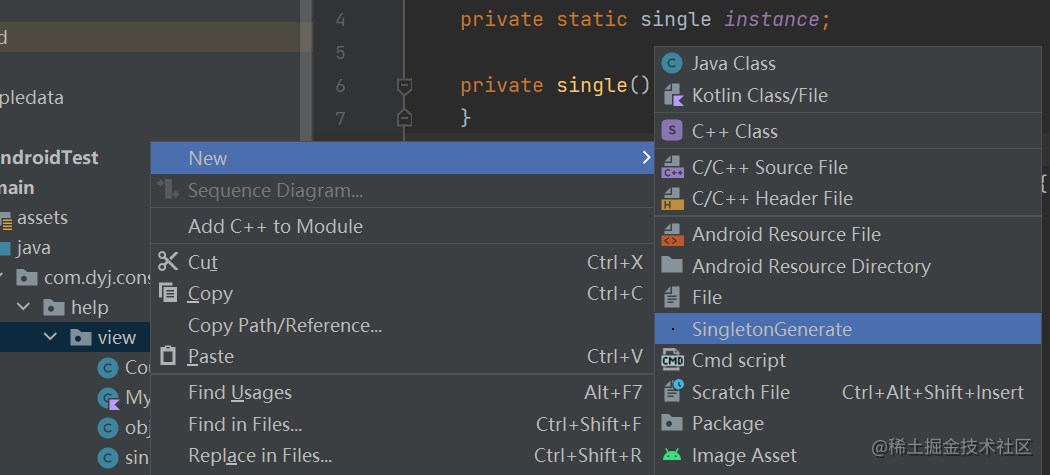21 0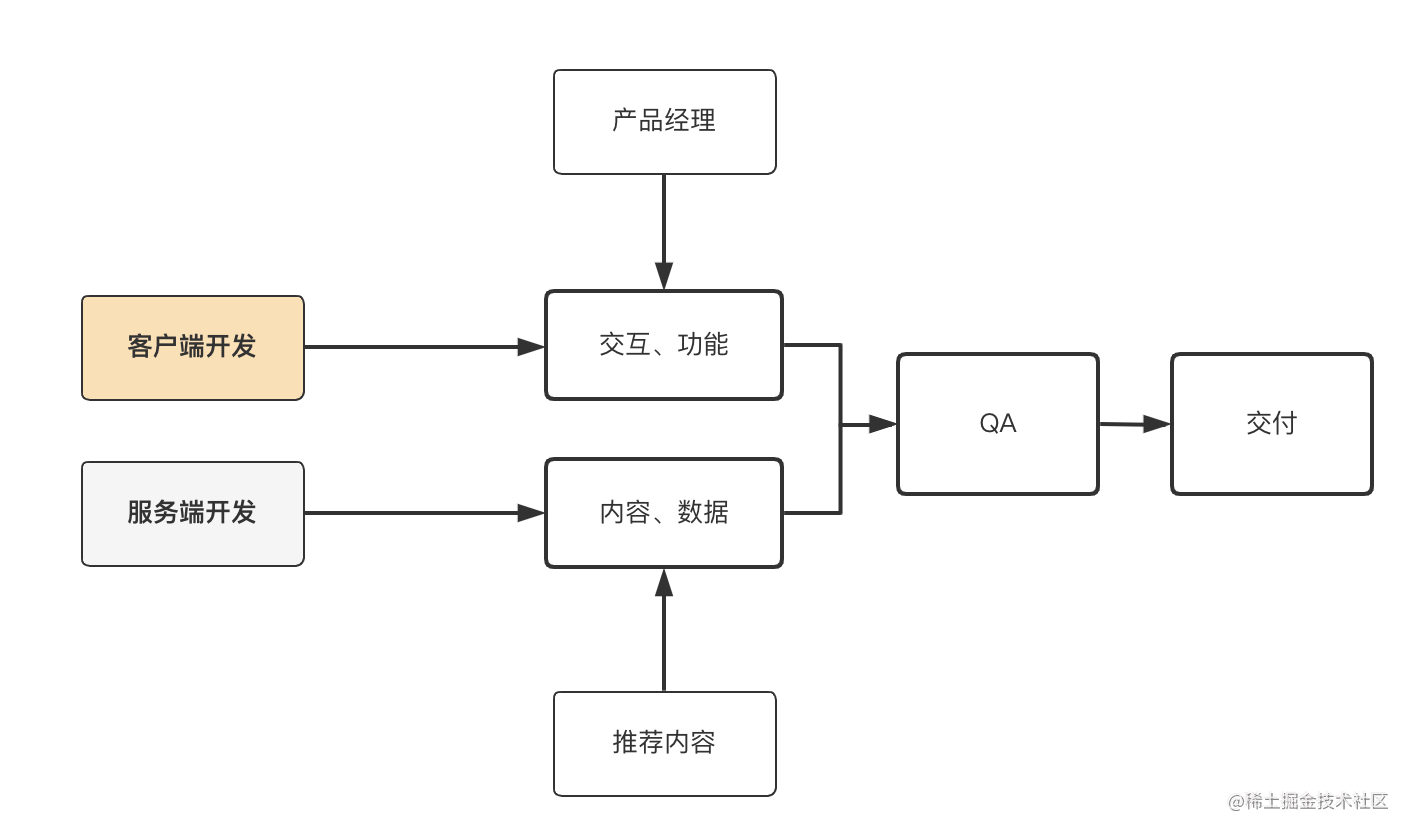41 0445 0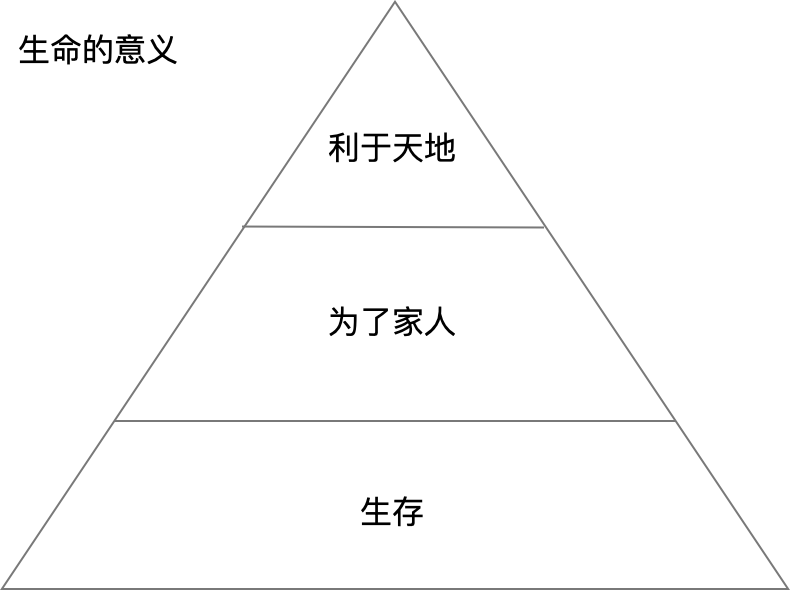431 0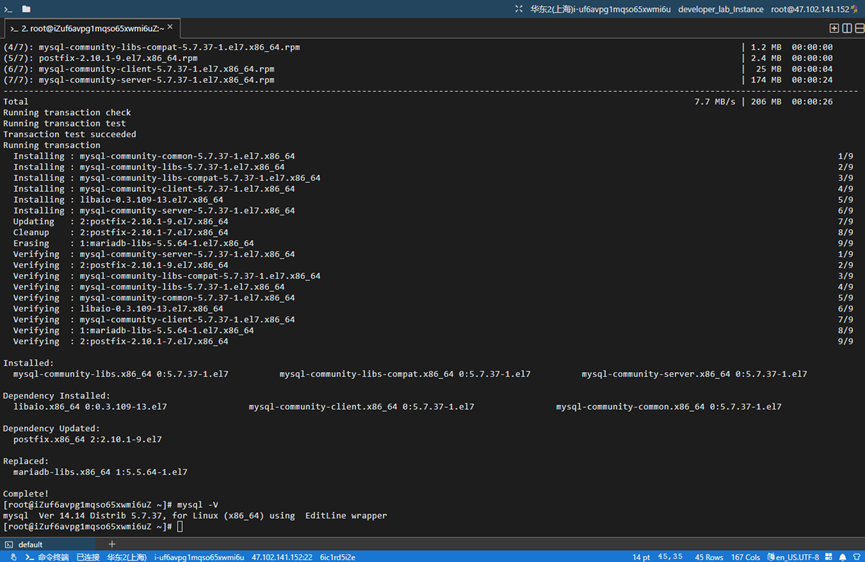56 0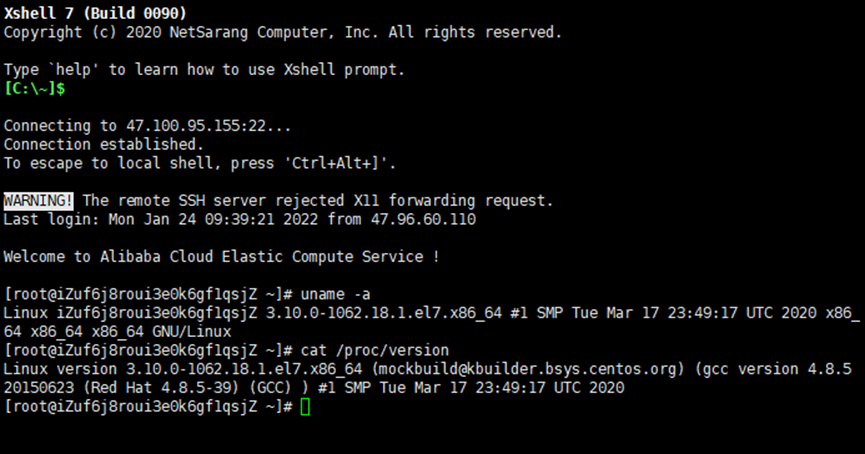62 01575 01195 0827 01533944

C囂冱僥楼縮殻189591285510242781846

Python点恰糞媾389086